# RD Sharma Solutions for Class 7 Maths Chapter 11 Percentage

In order to score high in Maths, RD Sharma Solutions is the best study material for the students. These materials can be downloaded easily by clicking the links provided here. The subject matter experts at BYJU’S have prepared the RD Sharma Solutions to help the students who are finding difficulties in solving them. Chapter 11, Percentage includes six exercises. Students can easily access answers to the problems present in RD Sharma Solutions for Class 7. Let us have a look at some of the important concepts that are being discussed in this Chapter.

• Meaning of percent
• Percent as a fraction
• Percent as a ratio
• Conversion of a percent into a fraction
• Conversion of fraction into a percent
• Conversion of ratio into percent and vice versa
• Conversion of a percent into decimal and vice versa
• Finding a percentage of a given number
• Some word problems on percentage

## Download the PDF of RD Sharma Solutions For Class 7 Maths Chapter 11 Percentage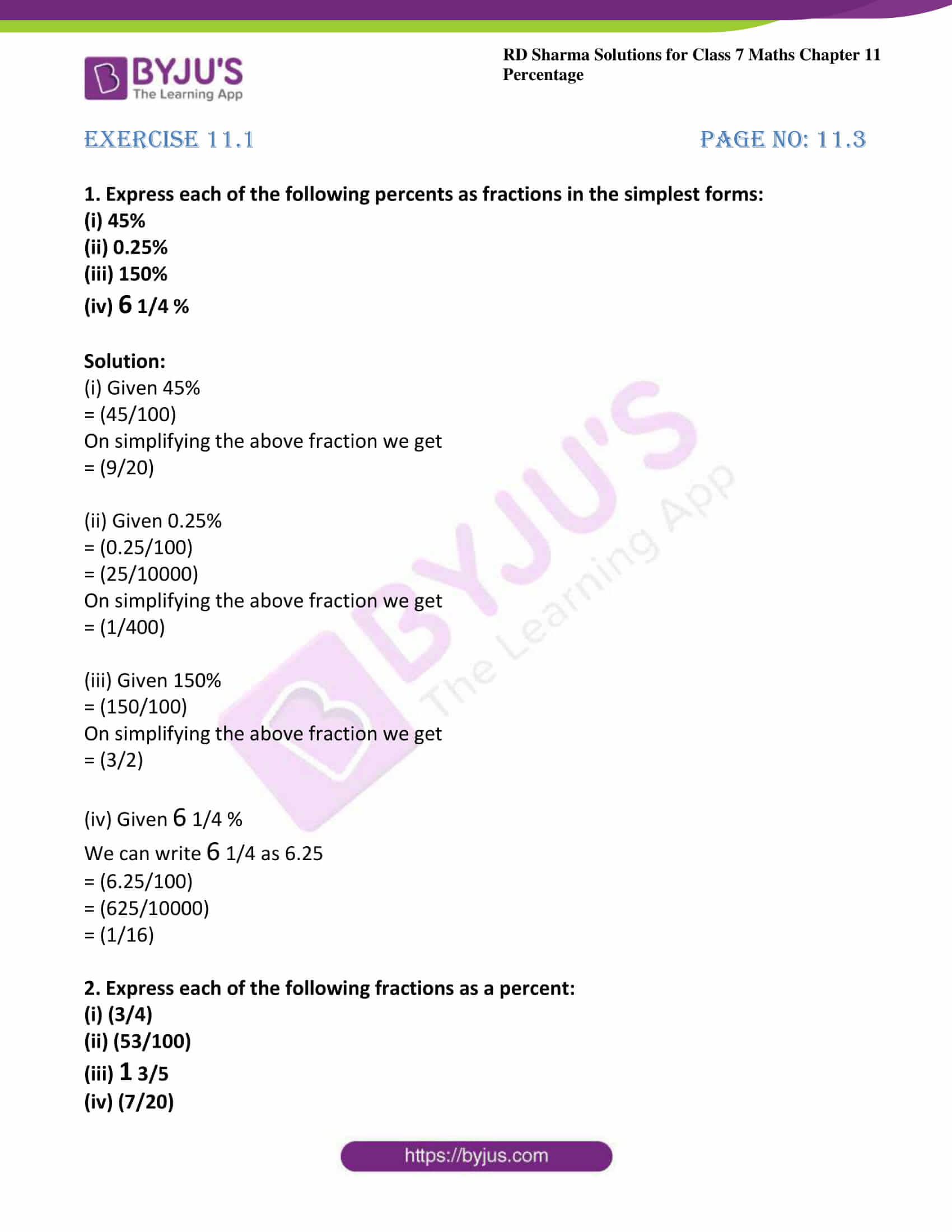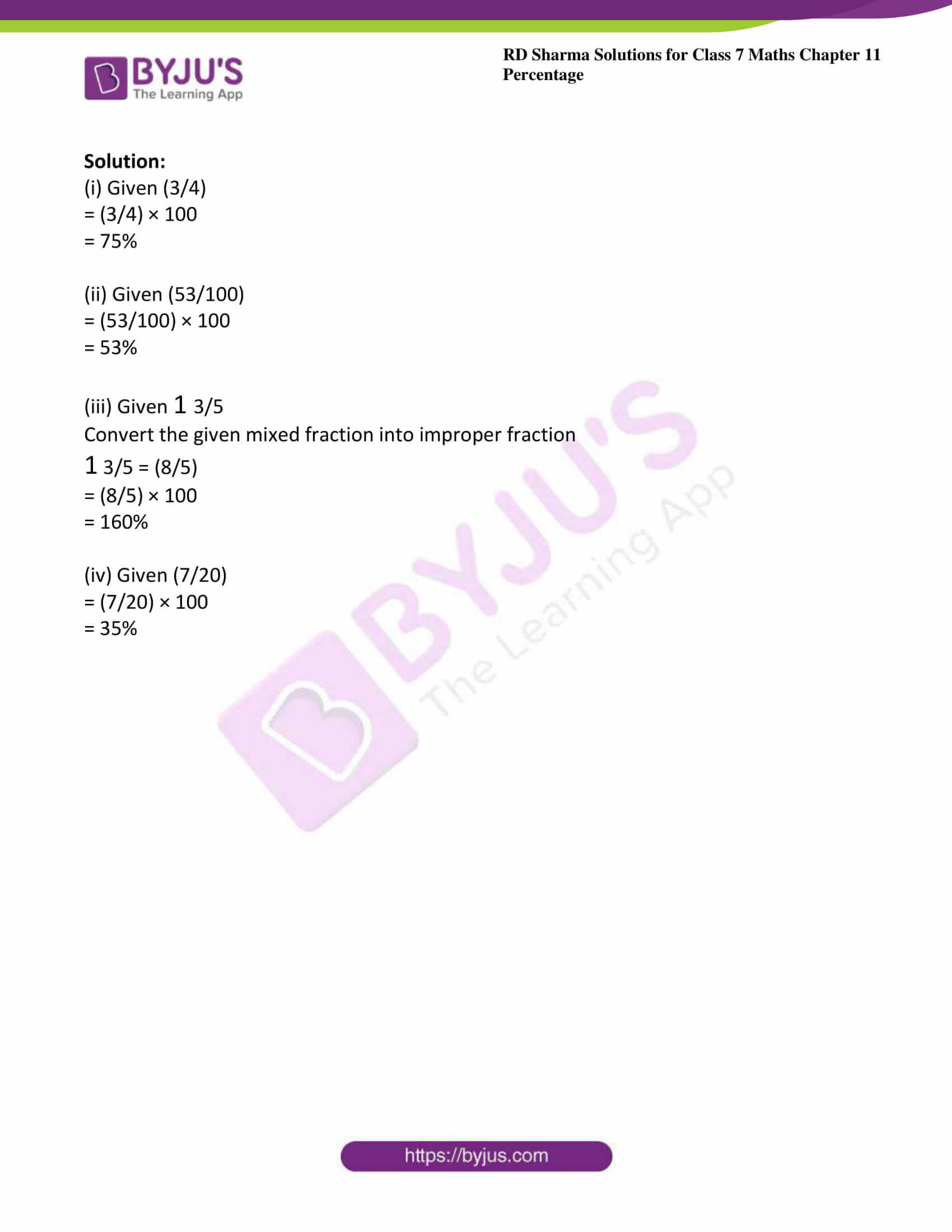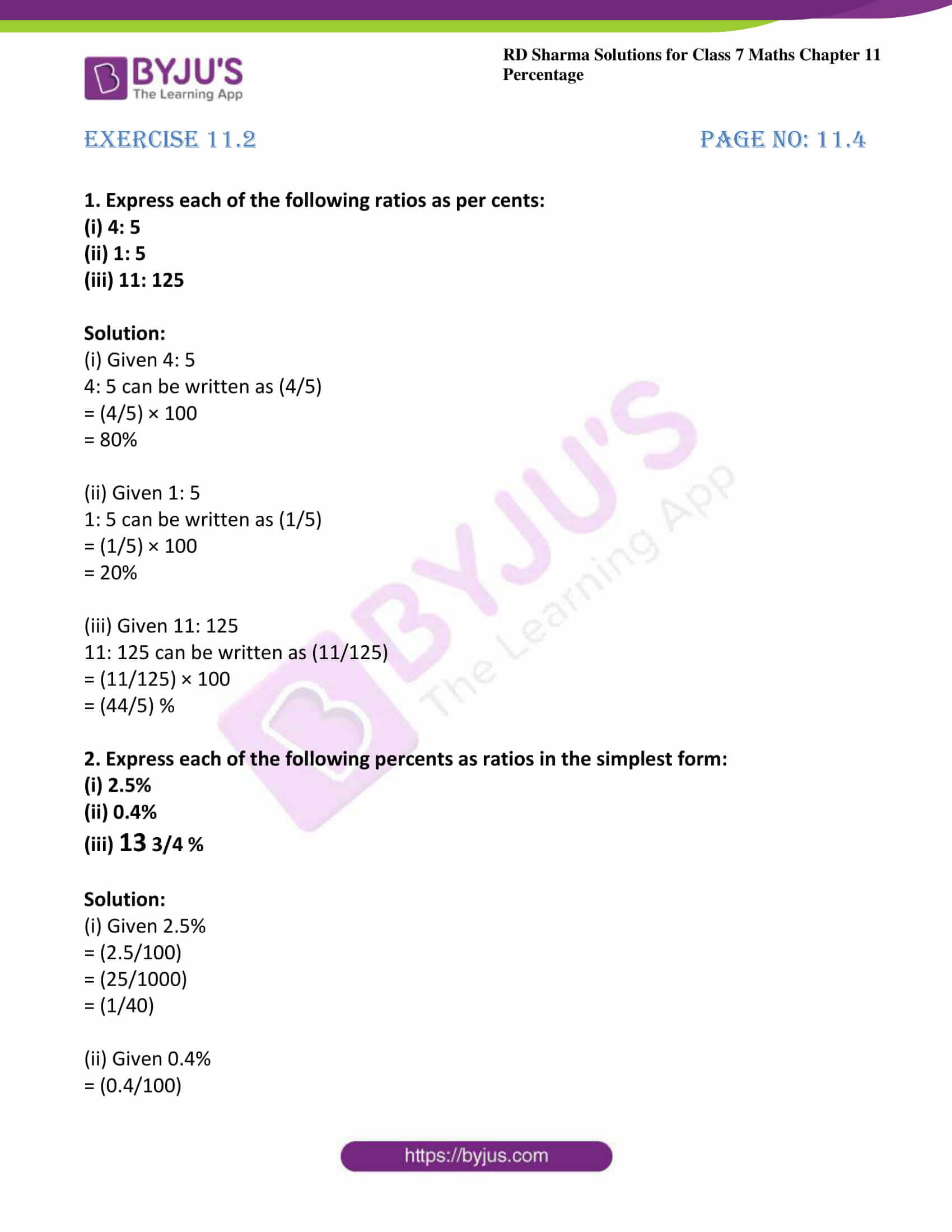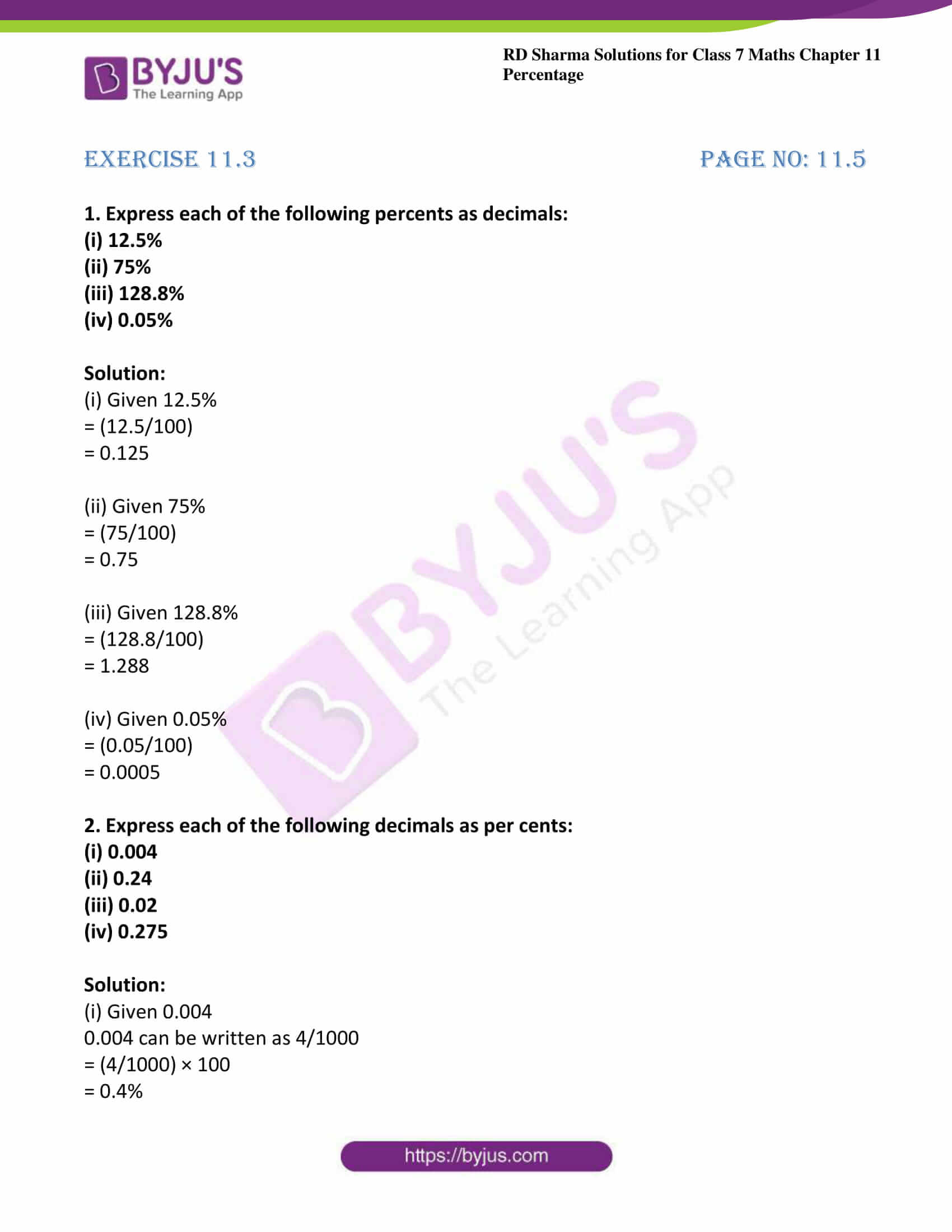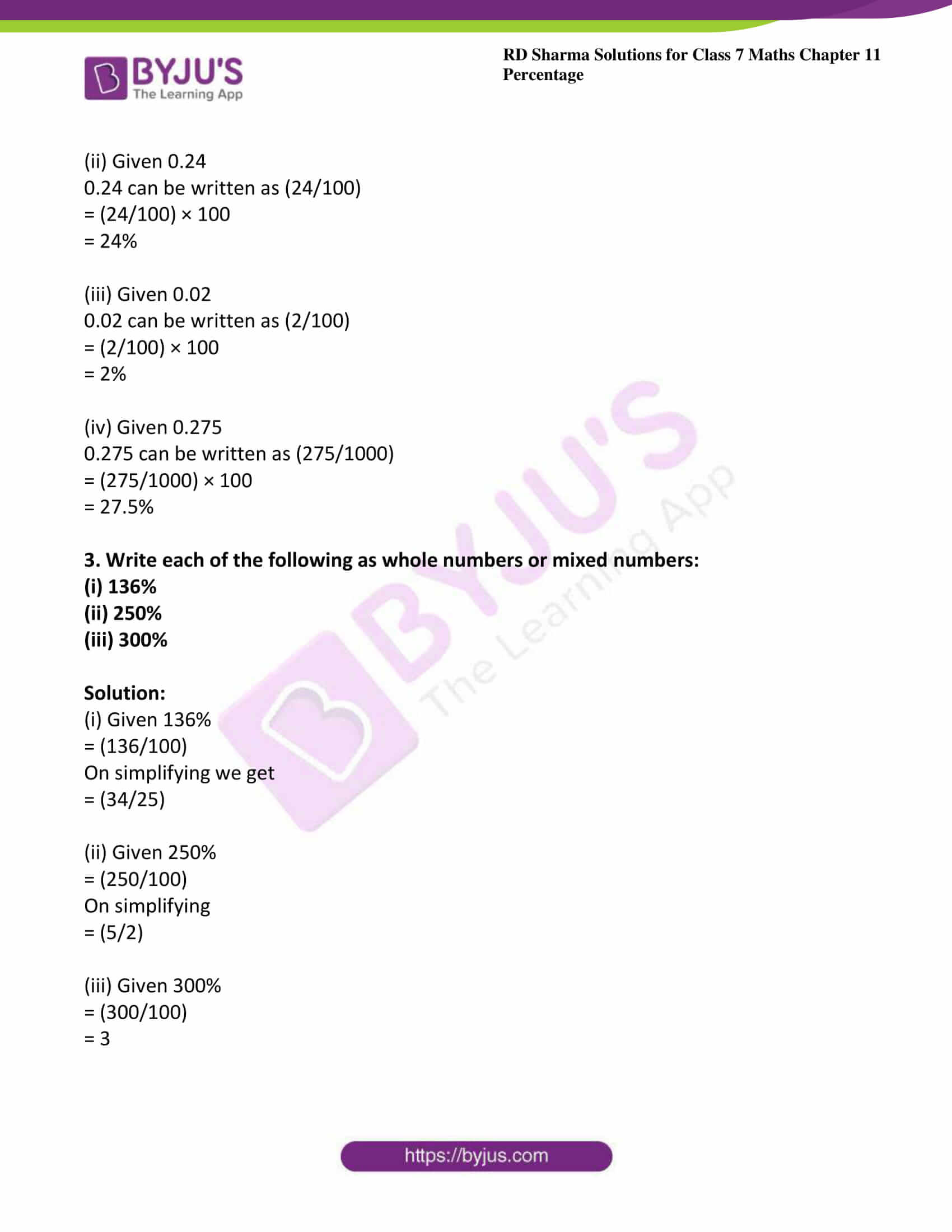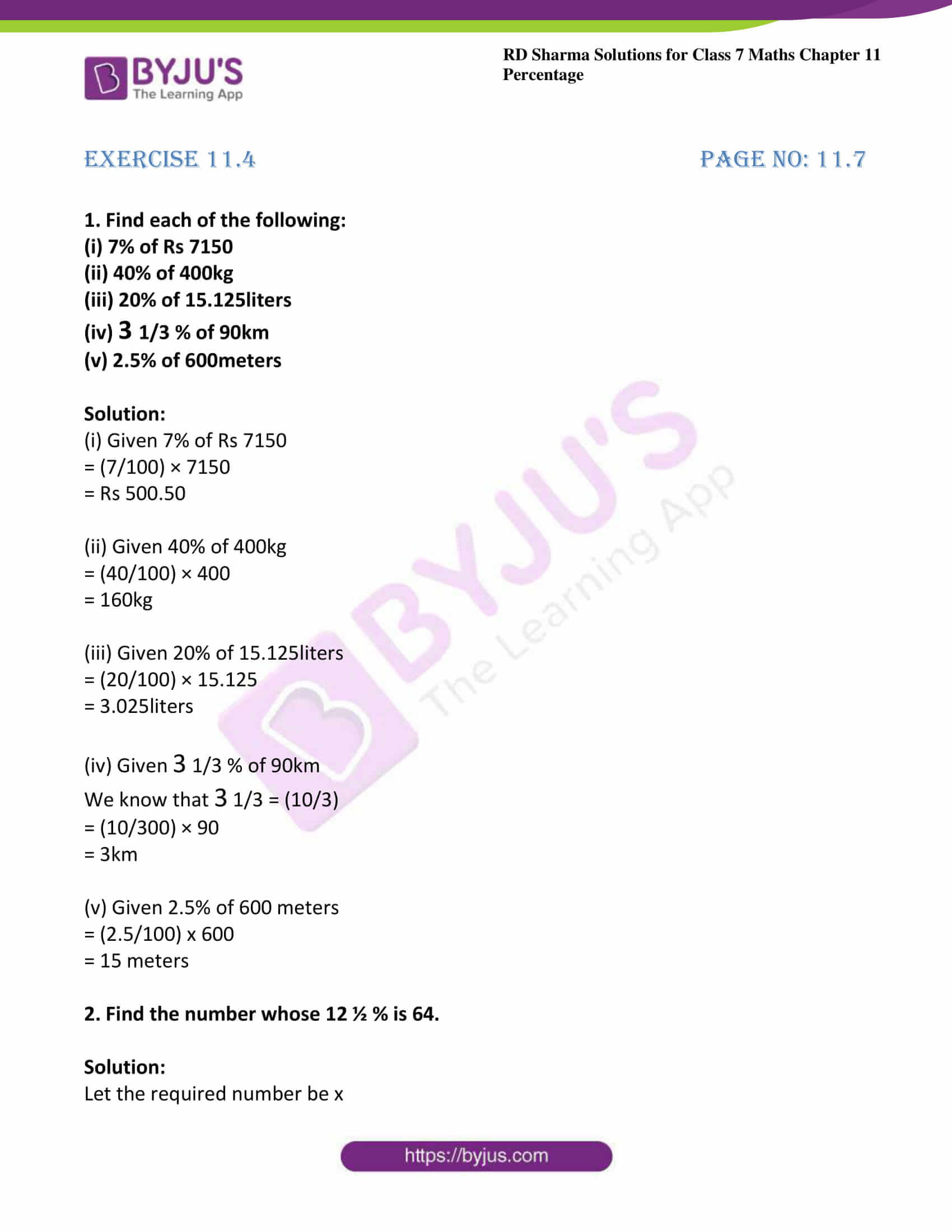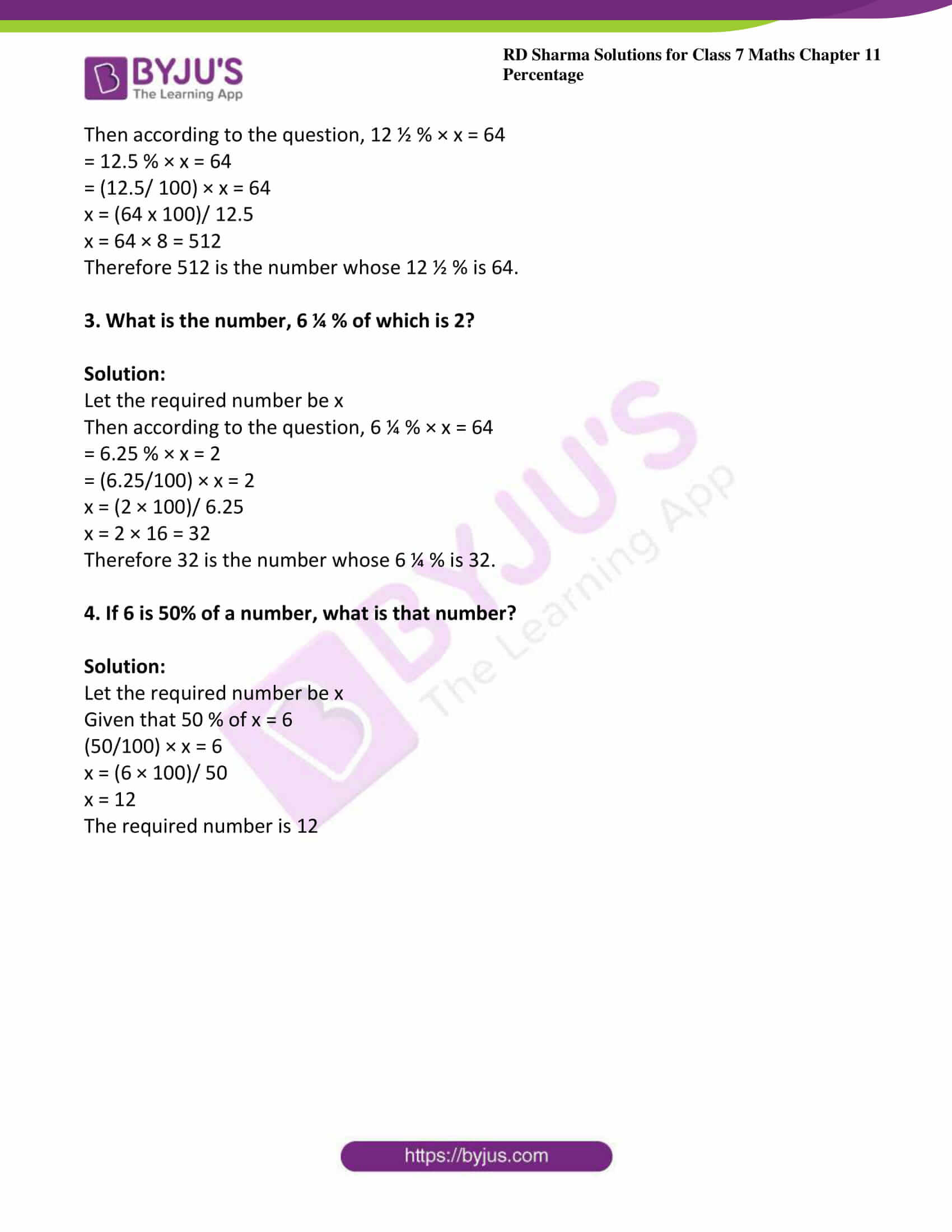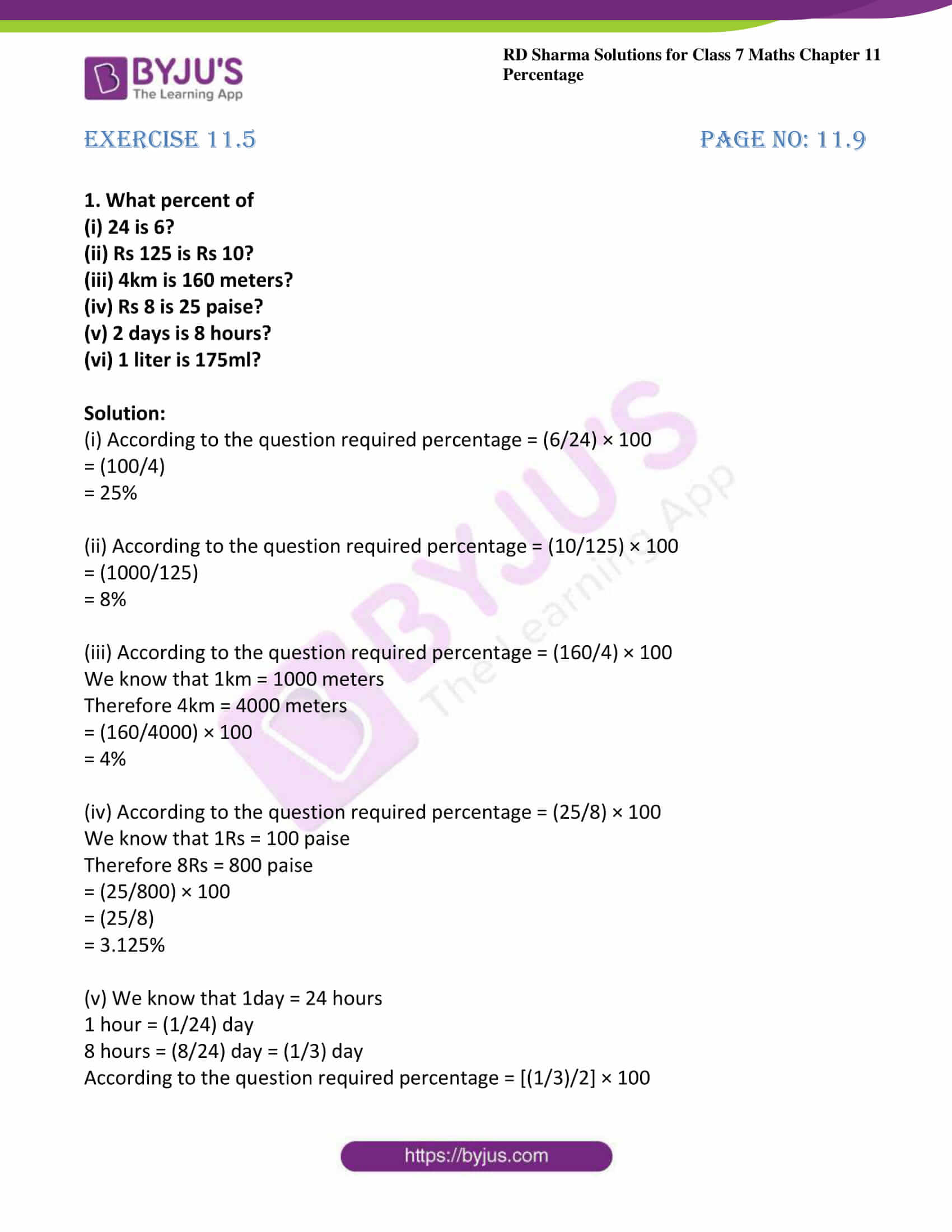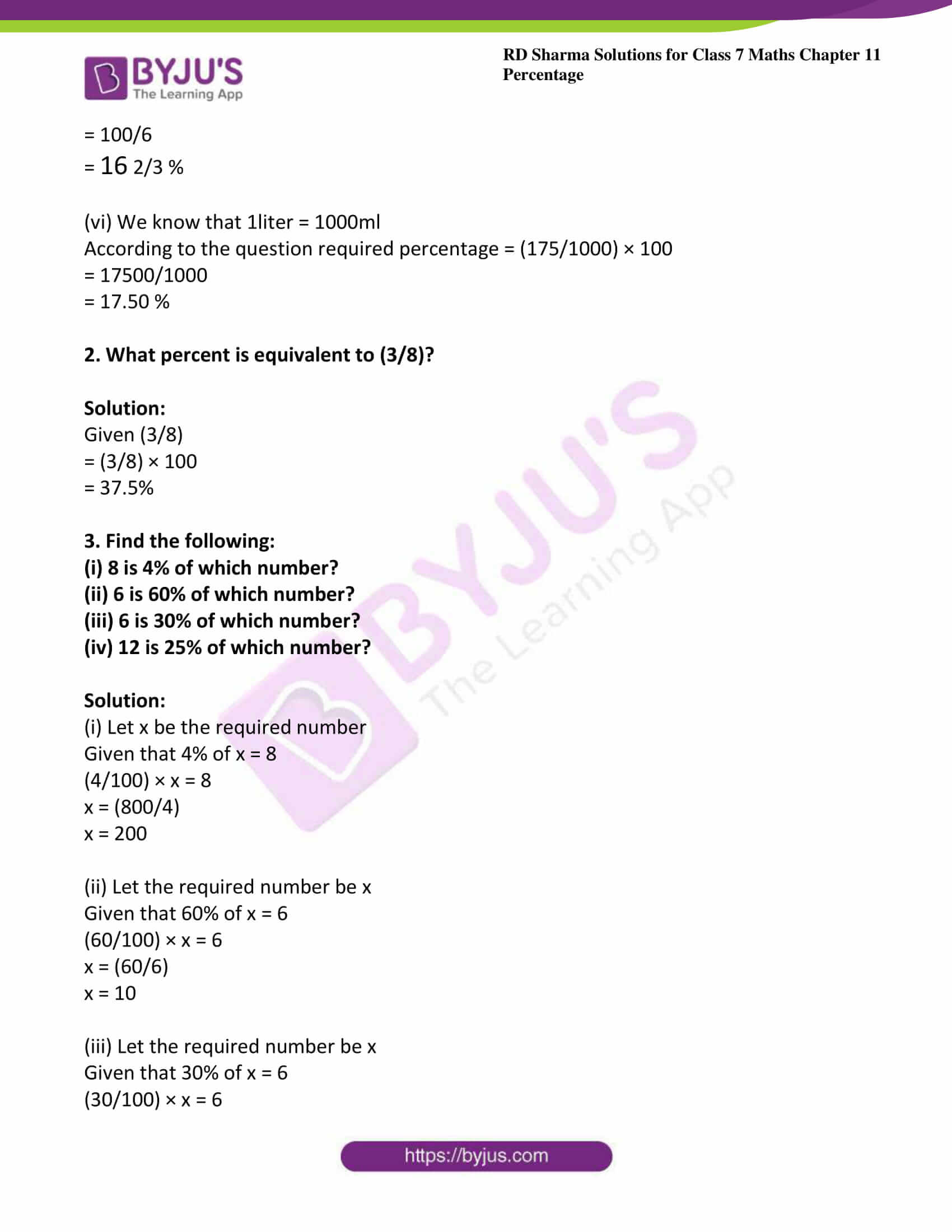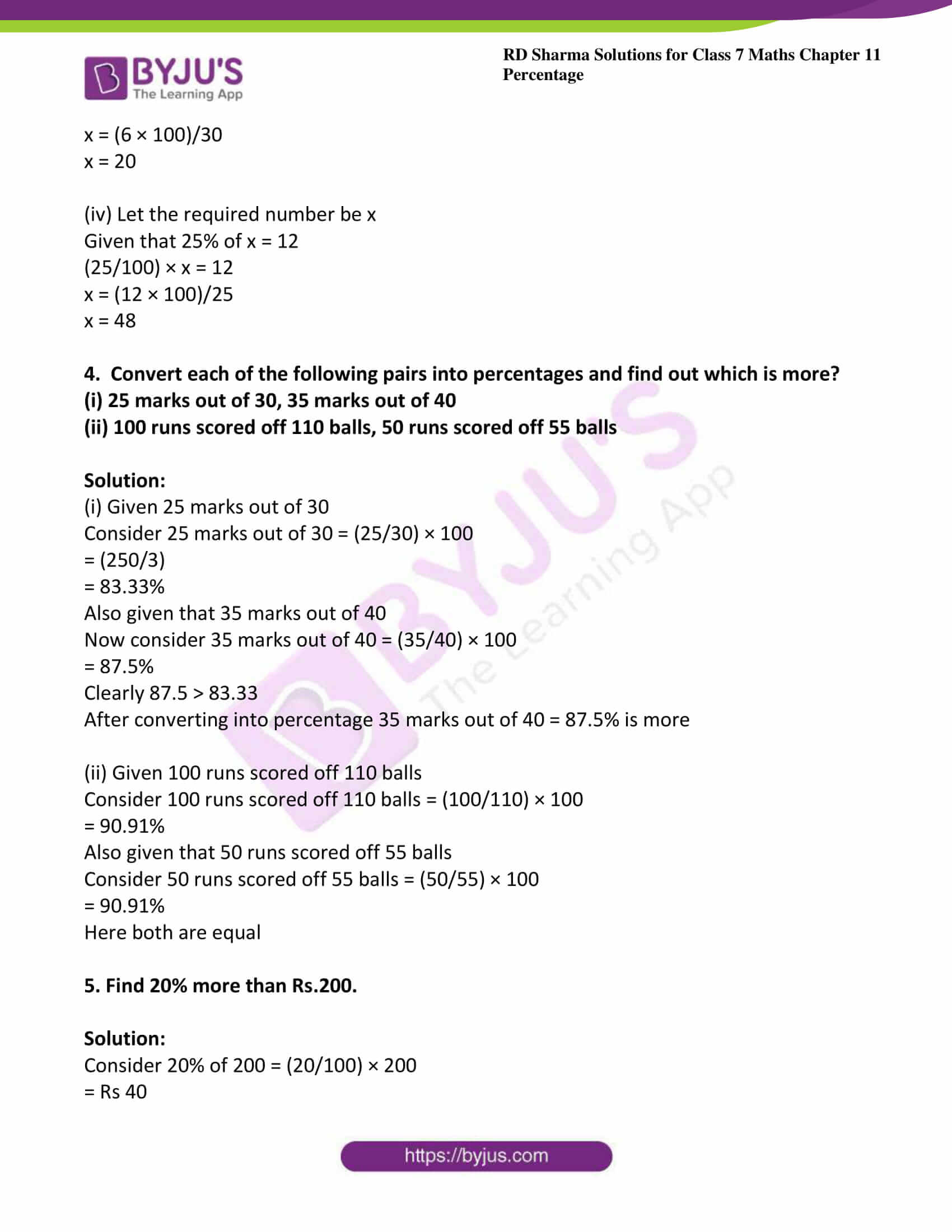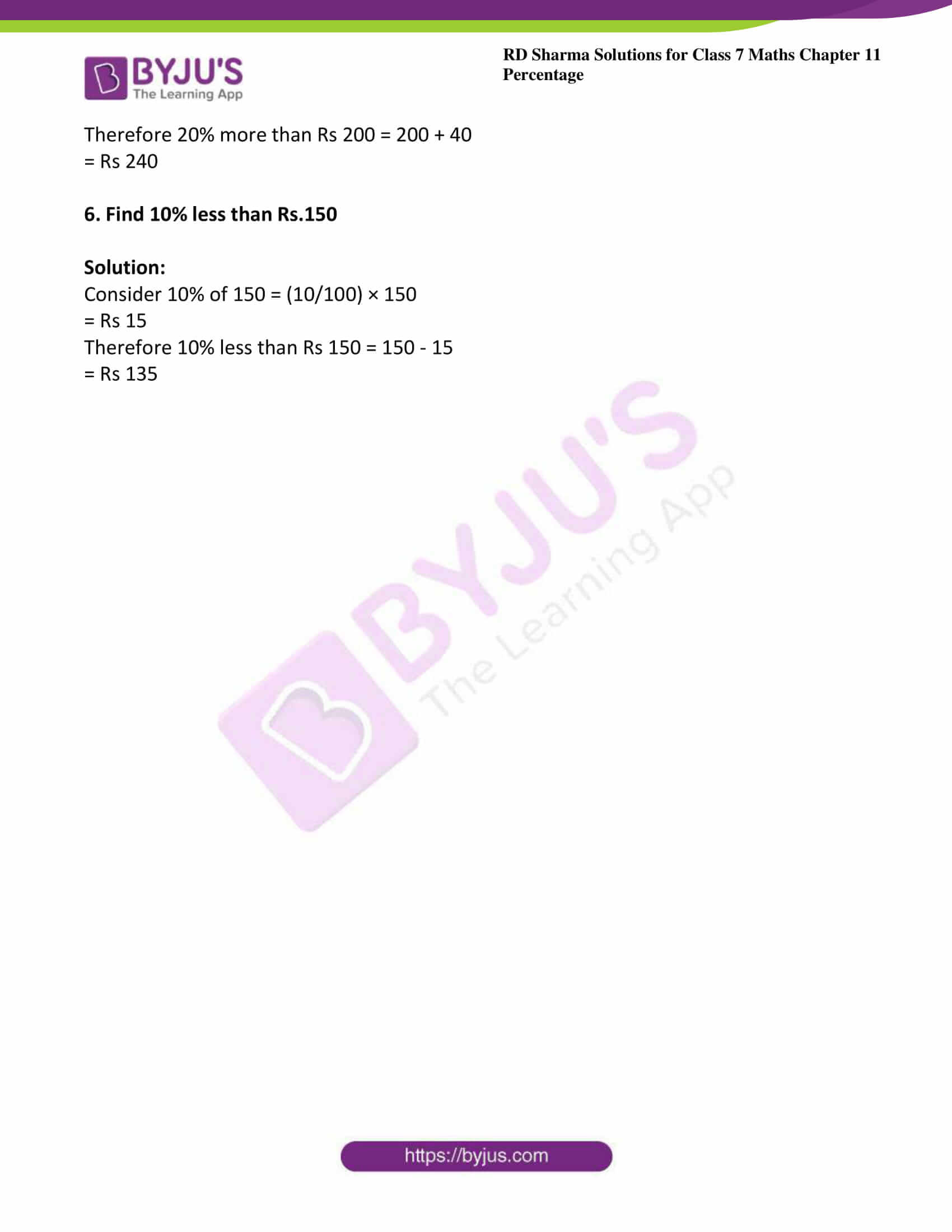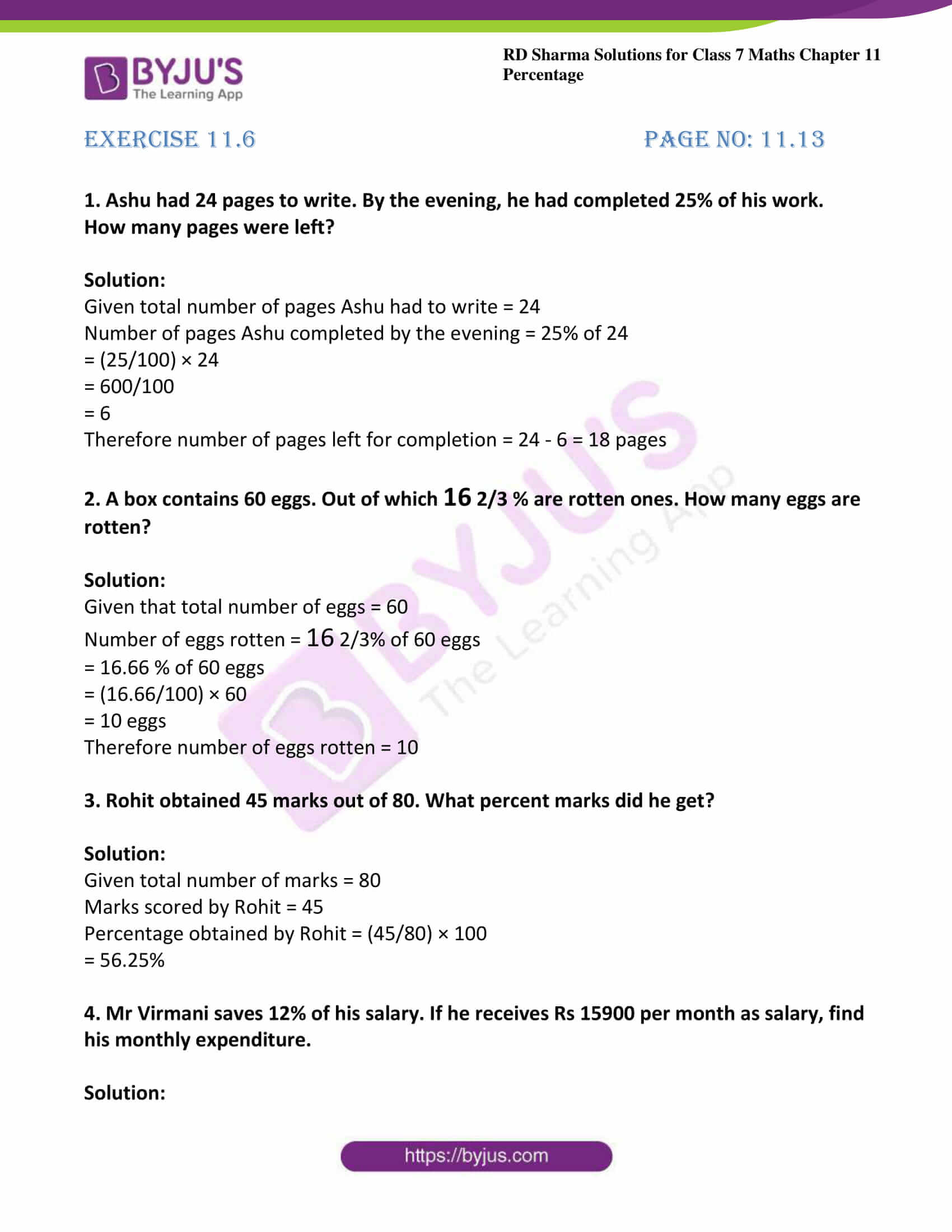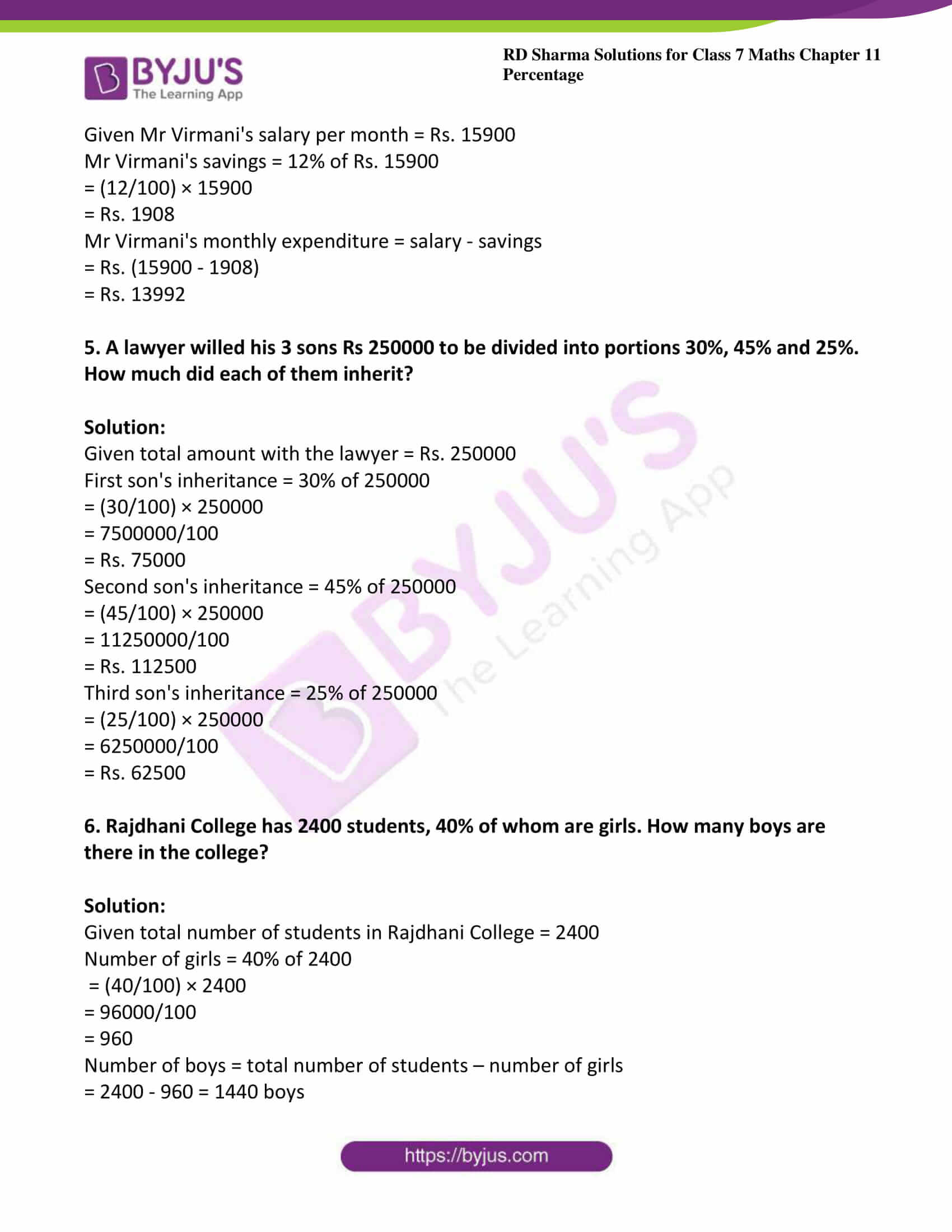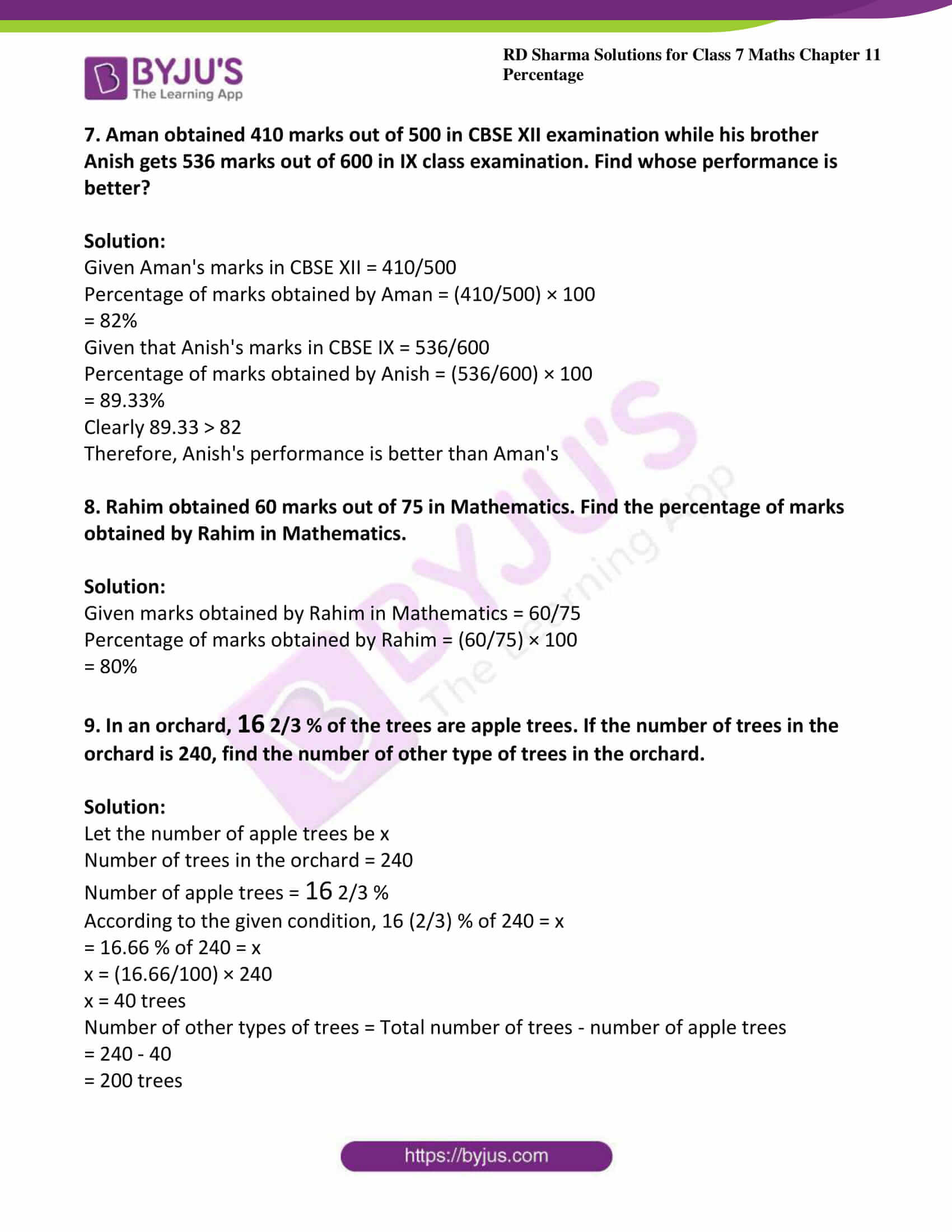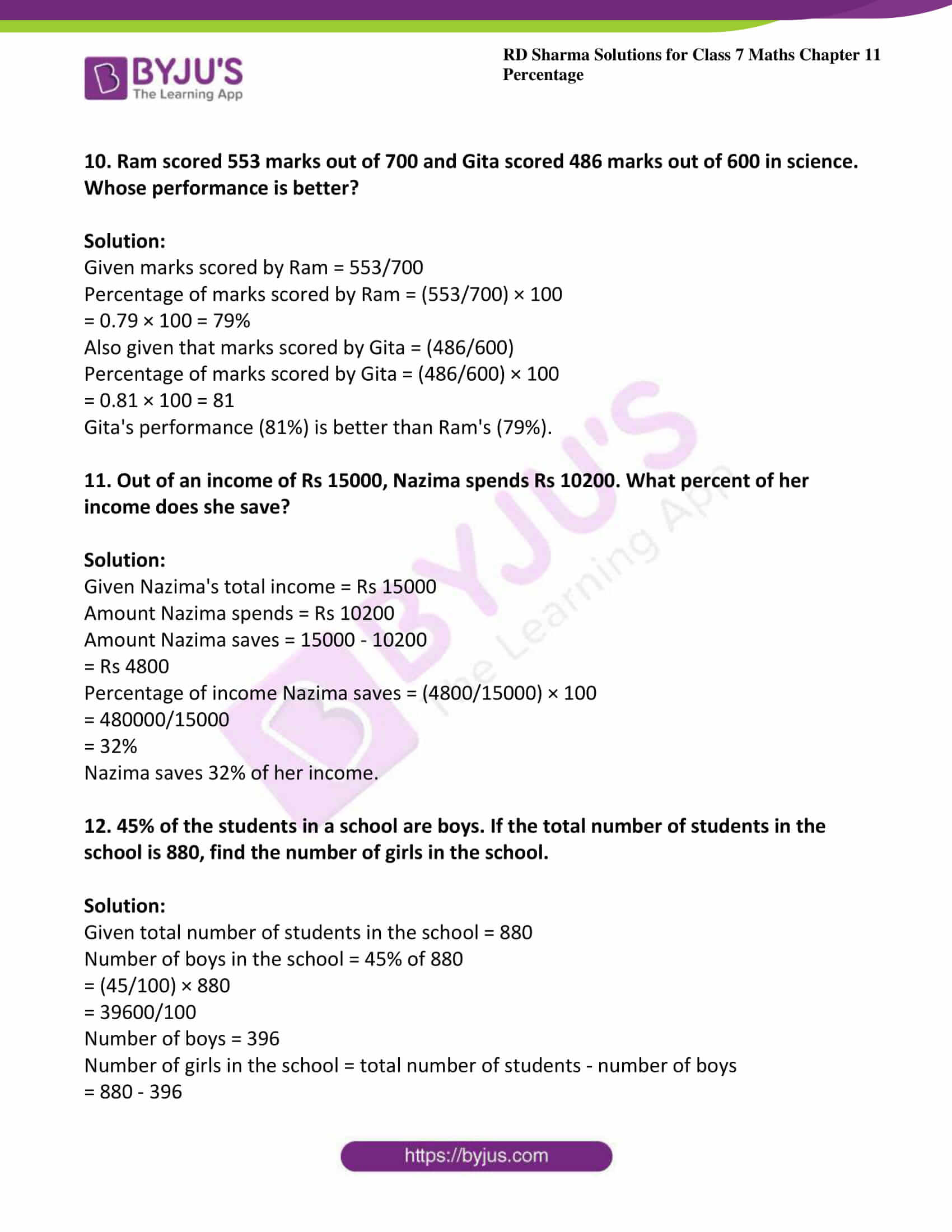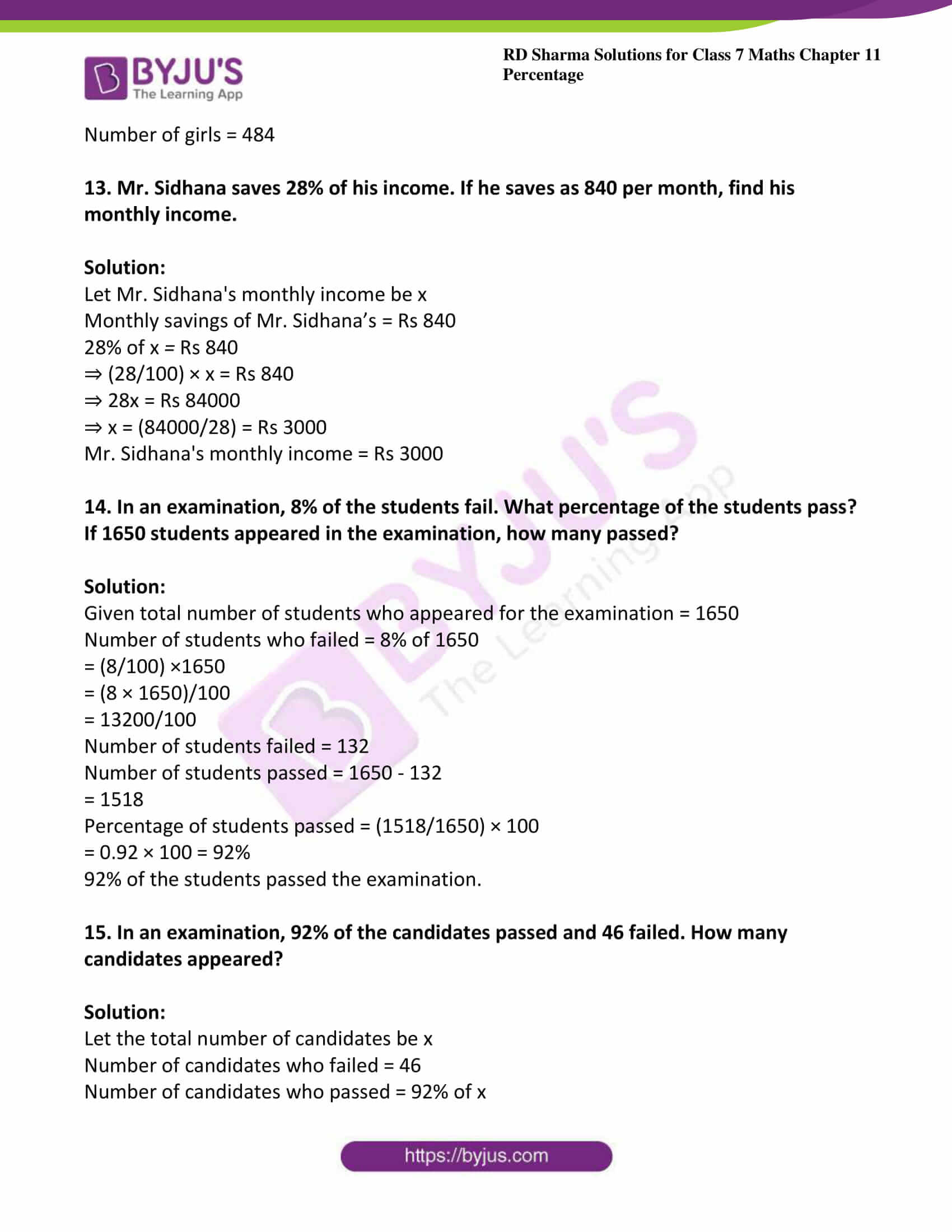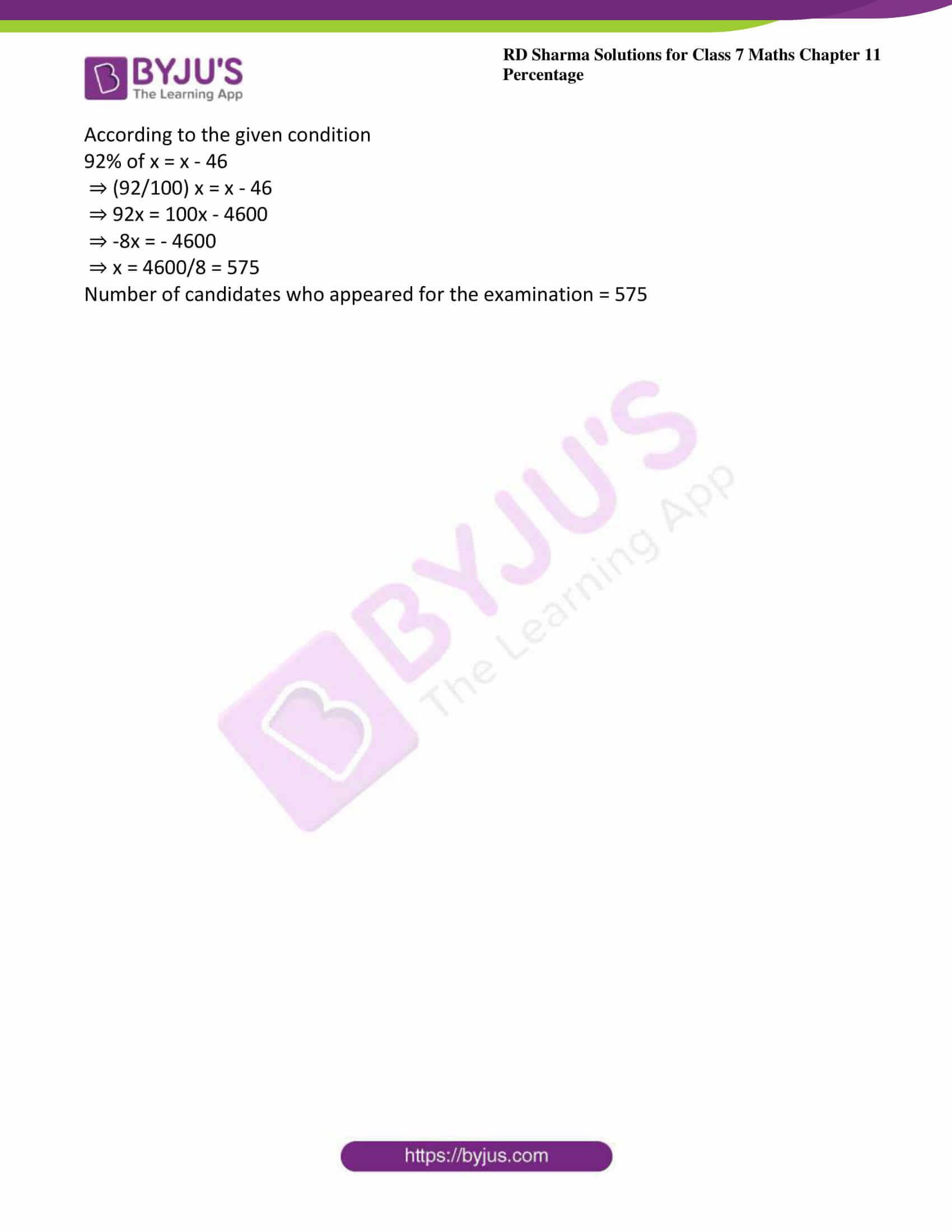### Access answers to Maths RD Sharma Solutions For Class 7 Chapter 11 – Percentage

Exercise 11.1 Page No: 11.3

1. Express each of the following percents as fractions in the simplest forms:

(i) 45%

(ii) 0.25%

(iii) 150%

(iv) 6 1/4 %

Solution:

(i) Given 45%

= (45/100)

On simplifying the above fraction we get

= (9/20)

(ii) Given 0.25%

= (0.25/100)

= (25/10000)

On simplifying the above fraction we get

= (1/400)

(iii) Given 150%

= (150/100)

On simplifying the above fraction we get

= (3/2)

(iv) Given 6 1/4 %

We can write 6 1/4 as 6.25

= (6.25/100)

= (625/10000)

= (1/16)

2. Express each of the following fractions as a percent:

(i) (3/4)

(ii) (53/100)

(iii) 1 3/5

(iv) (7/20)

Solution:

(i) Given (3/4)

= (3/4) × 100

= 75%

(ii) Given (53/100)

= (53/100) × 100

= 53%

(iii) Given 1 3/5

Convert the given mixed fraction into improper fraction

1 3/5 = (8/5)

= (8/5) × 100

= 160%

(iv) Given (7/20)

= (7/20) × 100

= 35%

Exercise 11.2 Page No: 11.4

1. Express each of the following ratios as per cents:

(i) 4: 5

(ii) 1: 5

(iii) 11: 125

Solution:

(i) Given 4: 5

4: 5 can be written as (4/5)

= (4/5) × 100

= 80%

(ii) Given 1: 5

1: 5 can be written as (1/5)

= (1/5) × 100

= 20%

(iii) Given 11: 125

11: 125 can be written as (11/125)

= (11/125) × 100

= (44/5) %

2. Express each of the following percents as ratios in the simplest form:

(i) 2.5%

(ii) 0.4%

(iii) 13 3/4 %

Solution:

(i) Given 2.5%

= (2.5/100)

= (25/1000)

= (1/40)

(ii) Given 0.4%

= (0.4/100)

= (4/1000)

= (1/250)

(iii) Given 13 3/4 %

13 3/4 = 13.75

= 13.75/100

= 1375/10000

= 11/80

Exercise 11.3 Page No: 11.5

1. Express each of the following percents as decimals:

(i) 12.5%

(ii) 75%

(iii) 128.8%

(iv) 0.05%

Solution:

(i) Given 12.5%

= (12.5/100)

= 0.125

(ii) Given 75%

= (75/100)

= 0.75

(iii) Given 128.8%

= (128.8/100)

= 1.288

(iv) Given 0.05%

= (0.05/100)

= 0.0005

2. Express each of the following decimals as per cents:

(i) 0.004

(ii) 0.24

(iii) 0.02

(iv) 0.275

Solution:

(i) Given 0.004

0.004 can be written as 4/1000

= (4/1000) × 100

= 0.4%

(ii) Given 0.24

0.24 can be written as (24/100)

= (24/100) × 100

= 24%

(iii) Given 0.02

0.02 can be written as (2/100)

= (2/100) × 100

= 2%

(iv) Given 0.275

0.275 can be written as (275/1000)

= (275/1000) × 100

= 27.5%

3. Write each of the following as whole numbers or mixed numbers:

(i) 136%

(ii) 250%

(iii) 300%

Solution:

(i) Given 136%

= (136/100)

On simplifying we get

= (34/25)

(ii) Given 250%

= (250/100)

On simplifying

= (5/2)

(iii) Given 300%

= (300/100)

= 3

Exercise 11.4 Page No: 11.7

1. Find each of the following:

(i) 7% of Rs 7150

(ii) 40% of 400kg

(iii) 20% of 15.125liters

(iv) 3 1/3 % of 90km

(v) 2.5% of 600meters

Solution:

(i) Given 7% of Rs 7150

= (7/100) × 7150

= Rs 500.50

(ii) Given 40% of 400kg

= (40/100) × 400

= 160kg

(iii) Given 20% of 15.125liters

= (20/100) × 15.125

= 3.025liters

(iv) Given 3 1/3 % of 90km

We know that 3 1/3 = (10/3)

= (10/300) × 90

= 3km

(v) Given 2.5% of 600 meters

= (2.5/100) x 600

= 15 meters

2. Find the number whose 12 ½ % is 64.

Solution:

Let the required number be x

Then according to the question, 12 ½ % × x = 64

= 12.5 % × x = 64

= (12.5/ 100) × x = 64

x = (64 x 100)/ 12.5

x = 64 × 8 = 512

Therefore 512 is the number whose 12 ½ % is 64.

3. What is the number, 6 ¼ % of which is 2?

Solution:

Let the required number be x

Then according to the question, 6 ¼ % × x = 64

= 6.25 % × x = 2

= (6.25/100) × x = 2

x = (2 × 100)/ 6.25

x = 2 × 16 = 32

Therefore 32 is the number whose 6 ¼ % is 32.

4. If 6 is 50% of a number, what is that number?

Solution:

Let the required number be x

Given that 50 % of x = 6

(50/100) × x = 6

x = (6 × 100)/ 50

x = 12

The required number is 12

Exercise 11.5 Page No: 11.9

1. What percent of

(i) 24 is 6?

(ii) Rs 125 is Rs 10?

(iii) 4km is 160 meters?

(iv) Rs 8 is 25 paise?

(v) 2 days is 8 hours?

(vi) 1 liter is 175ml?

Solution:

(i) According to the question required percentage = (6/24) × 100

= (100/4)

= 25%

(ii) According to the question required percentage = (10/125) × 100

= (1000/125)

= 8%

(iii) According to the question required percentage = (160/4) × 100

We know that 1km = 1000 meters

Therefore 4km = 4000 meters

= (160/4000) × 100

= 4%

(iv) According to the question required percentage = (25/8) × 100

We know that 1Rs = 100 paise

Therefore 8Rs = 800 paise

= (25/800) × 100

= (25/8)

= 3.125%

(v) We know that 1day = 24 hours

1 hour = (1/24) day

8 hours = (8/24) day = (1/3) day

According to the question required percentage = [(1/3)/2] × 100

= 100/6

= 16 2/3 %

(vi) We know that 1liter = 1000ml

According to the question required percentage = (175/1000) × 100

= 17500/1000

= 17.50 %

2. What percent is equivalent to (3/8)?

Solution:

Given (3/8)

= (3/8) × 100

= 37.5%

3. Find the following:

(i) 8 is 4% of which number?

(ii) 6 is 60% of which number?

(iii) 6 is 30% of which number?

(iv) 12 is 25% of which number?

Solution:

(i) Let x be the required number

Given that 4% of x = 8

(4/100) × x = 8

x = (800/4)

x = 200

(ii) Let the required number be x

Given that 60% of x = 6

(60/100) × x = 6

x = (60/6)

x = 10

(iii) Let the required number be x

Given that 30% of x = 6

(30/100) × x = 6

x = (6 × 100)/30

x = 20

(iv) Let the required number be x

Given that 25% of x = 12

(25/100) × x = 12

x = (12 × 100)/25

x = 48

4. Convert each of the following pairs into percentages and find out which is more?

(i) 25 marks out of 30, 35 marks out of 40

(ii) 100 runs scored off 110 balls, 50 runs scored off 55 balls

Solution:

(i) Given 25 marks out of 30

Consider 25 marks out of 30 = (25/30) × 100

= (250/3)

= 83.33%

Also given that 35 marks out of 40

Now consider 35 marks out of 40 = (35/40) × 100

= 87.5%

Clearly 87.5 > 83.33

After converting into percentage 35 marks out of 40 = 87.5% is more

(ii) Given 100 runs scored off 110 balls

Consider 100 runs scored off 110 balls = (100/110) × 100

= 90.91%

Also given that 50 runs scored off 55 balls

Consider 50 runs scored off 55 balls = (50/55) × 100

= 90.91%

Here both are equal

5. Find 20% more than Rs.200.

Solution:

Consider 20% of 200 = (20/100) × 200

= Rs 40

Therefore 20% more than Rs 200 = 200 + 40

= Rs 240

6. Find 10% less than Rs.150

Solution:

Consider 10% of 150 = (10/100) × 150

= Rs 15

Therefore 10% less than Rs 150 = 150 – 15

= Rs 135

Exercise 11.6 Page No: 11.13

1. Ashu had 24 pages to write. By the evening, he had completed 25% of his work. How many pages were left?

Solution:

Given total number of pages Ashu had to write = 24

Number of pages Ashu completed by the evening = 25% of 24

= (25/100) × 24

= 600/100

= 6

Therefore number of pages left for completion = 24 – 6 = 18 pages

2. A box contains 60 eggs. Out of which 16 2/3 % are rotten ones. How many eggs are rotten?

Solution:

Given that total number of eggs = 60

Number of eggs rotten = 16 2/3% of 60 eggs

= 16.66 % of 60 eggs

= (16.66/100) × 60

= 10 eggs

Therefore number of eggs rotten = 10

3. Rohit obtained 45 marks out of 80. What percent marks did he get?

Solution:

Given total number of marks = 80

Marks scored by Rohit = 45

Percentage obtained by Rohit = (45/80) × 100

= 56.25%

4. Mr Virmani saves 12% of his salary. If he receives Rs 15900 per month as salary, find his monthly expenditure.

Solution:

Given Mr Virmani’s salary per month = Rs. 15900

Mr Virmani’s savings = 12% of Rs. 15900

= (12/100) × 15900

= Rs. 1908

Mr Virmani’s monthly expenditure = salary – savings

= Rs. (15900 – 1908)

= Rs. 13992

5. A lawyer willed his 3 sons Rs 250000 to be divided into portions 30%, 45% and 25%. How much did each of them inherit?

Solution:

Given total amount with the lawyer = Rs. 250000

First son’s inheritance = 30% of 250000

= (30/100) × 250000

= 7500000/100

= Rs. 75000

Second son’s inheritance = 45% of 250000

= (45/100)​ × 250000

= 11250000/100

= Rs. 112500

Third son’s inheritance = 25% of 250000

= (25/100)​ × 250000

= 6250000/100

= Rs. 62500

6. Rajdhani College has 2400 students, 40% of whom are girls. How many boys are there in the college?

Solution:

Given total number of students in Rajdhani College = 2400

Number of girls = 40% of 2400

= (40/100) × 2400

= 96000/100

= 960

Number of boys = total number of students – number of girls

= 2400 – 960 = 1440 boys

7. Aman obtained 410 marks out of 500 in CBSE XII examination while his brother Anish gets 536 marks out of 600 in IX class examination. Find whose performance is better?

Solution:

Given Aman’s marks in CBSE XII = 410/500

Percentage of marks obtained by Aman = (410/500) × 100

= 82%

Given that Anish’s marks in CBSE IX = 536/600

Percentage of marks obtained by Anish = (536/600) × 100

= 89.33%

Clearly 89.33 > 82

Therefore, Anish’s performance is better than Aman’s

8. Rahim obtained 60 marks out of 75 in Mathematics. Find the percentage of marks obtained by Rahim in Mathematics.

Solution:

Given marks obtained by Rahim in Mathematics = 60/75

Percentage of marks obtained by Rahim = (60/75) × 100

= 80%

9. In an orchard, 16 2/3 % of the trees are apple trees. If the number of trees in the orchard is 240, find the number of other type of trees in the orchard.

Solution:

Let the number of apple trees be x

Number of trees in the orchard = 240

Number of apple trees = 16 2/3 %

According to the given condition, 16 (2/3) % of 240 = x

= 16.66 % of 240 = x

x = (16.66/100) × 240

x = 40 trees

Number of other types of trees = Total number of trees – number of apple trees

= 240 – 40

= 200 trees

10. Ram scored 553 marks out of 700 and Gita scored 486 marks out of 600 in science. Whose performance is better?

Solution:

Given marks scored by Ram = 553/700

Percentage of marks scored by Ram = (553/700) × 100

= 0.79 × 100 = 79%

Also given that marks scored by Gita = (486/600)

Percentage of marks scored by Gita = (486/600) × 100

= 0.81 × 100 = 81

Gita’s performance (81%) is better than Ram’s (79%).

11. Out of an income of Rs 15000, Nazima spends Rs 10200. What percent of her income does she save?

Solution:

Given Nazima’s total income = Rs 15000

Amount Nazima spends = Rs 10200

Amount Nazima saves = 15000 – 10200

= Rs 4800

Percentage of income Nazima saves = (4800/15000) × 100

= 480000/15000

= 32%

Nazima saves 32% of her income.

12. 45% of the students in a school are boys. If the total number of students in the school is 880, find the number of girls in the school.

Solution:

Given total number of students in the school = 880

Number of boys in the school = 45% of 880

= (45/100) × 880

= 39600/100

Number of boys = 396

Number of girls in the school = total number of students – number of boys

= 880 – 396

Number of girls = 484

13. Mr. Sidhana saves 28% of his income. If he saves as 840 per month, find his monthly income.

Solution:

Let Mr. Sidhana’s monthly income be x

Monthly savings of Mr. Sidhana’s = Rs 840

28% of xRs 840

⇒ (28/100) × x = Rs 840

⇒ 28x = Rs 84000

⇒ x = (84000/28) = Rs 3000

Mr. Sidhana’s monthly income = Rs 3000

14. In an examination, 8% of the students fail. What percentage of the students pass? If 1650 students appeared in the examination, how many passed?

Solution:

Given total number of students who appeared for the examination = 1650

Number of students who failed = 8% of 1650

= (8/100) ×1650

= (8 × 1650)/100

= 13200/100

Number of students failed = 132

Number of students passed = 1650 – 132

= 1518

Percentage of students passed = (1518/1650) × 100

= 0.92 × 100 = 92%

92% of the students passed the examination.

15. In an examination, 92% of the candidates passed and 46 failed. How many candidates appeared?

Solution:

Let the total number of candidates be x

Number of candidates who failed = 46

Number of candidates who passed = 92% of x

According to the given condition

92% of x = x – 46

⇒ (92/100) x = x – 46

⇒ 92x = 100x – 4600

⇒ -8x = – 4600

⇒ x = 4600/8 = 575

Number of candidates who appeared for the examination = 575